# 基于微分思想的层合材料传热问题新解法*

(华南理工大学广州学院， 广州 510800)

## 引 言

2010年盛宏玉、李和平等运用状态空间理论，建立了时域空间内的差分方程，分析了层合材料传热问题2011年蓝林华、富明慧等将层合材料的传热问题转化为在时间域内的常微分方程问题，给出了一种有效的解法2011年李金娥等对某一双层结构进行研究，建立了Fourier(傅里叶)和非Fourier层合材料传热差分方程，研究了其温度场和热应力情况2012年富明慧等利用半解析精细积分法求解了层合圆柱体的三维温度场2012年Liu等利用Laplace(拉普拉斯)变换的方法研究了三层结构传热的问题2014年Movahedian等利用指数函数法研究了层合材料的传热问题2016年Mohajer等利用数值方法研究了层合材料传热的非Fourier问题

## 1 模 型 建 立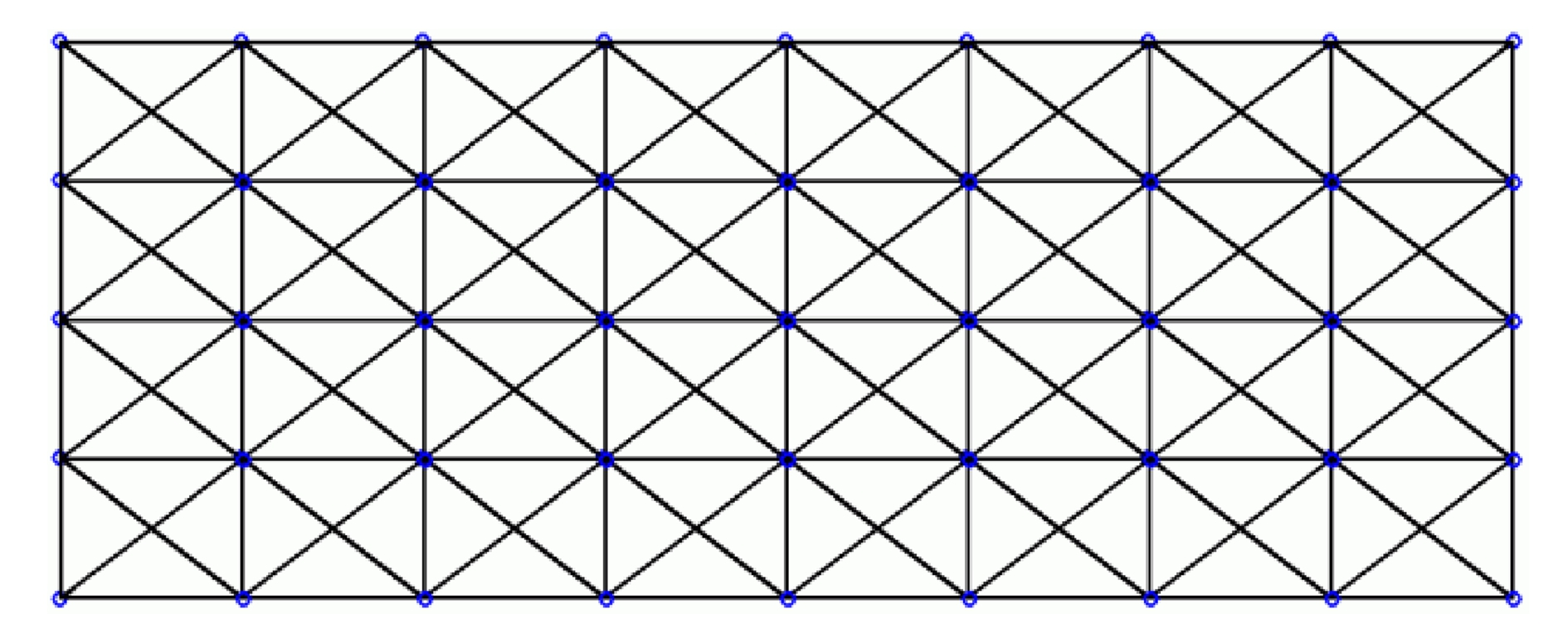Fig. 1 The geometrical model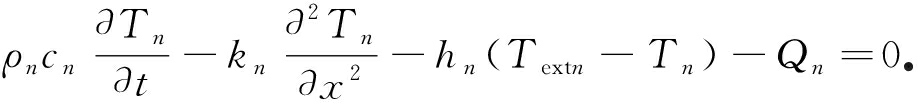(1)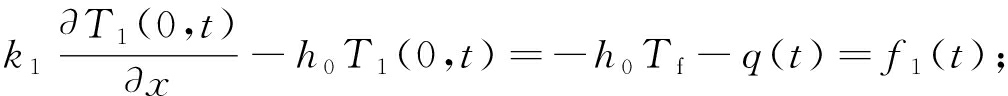(2)

x=ln

Tn(ln,t)=fn(t)=T0

(3)

Tn(x,0)=Tina

(4)

## 2 求 解 思 路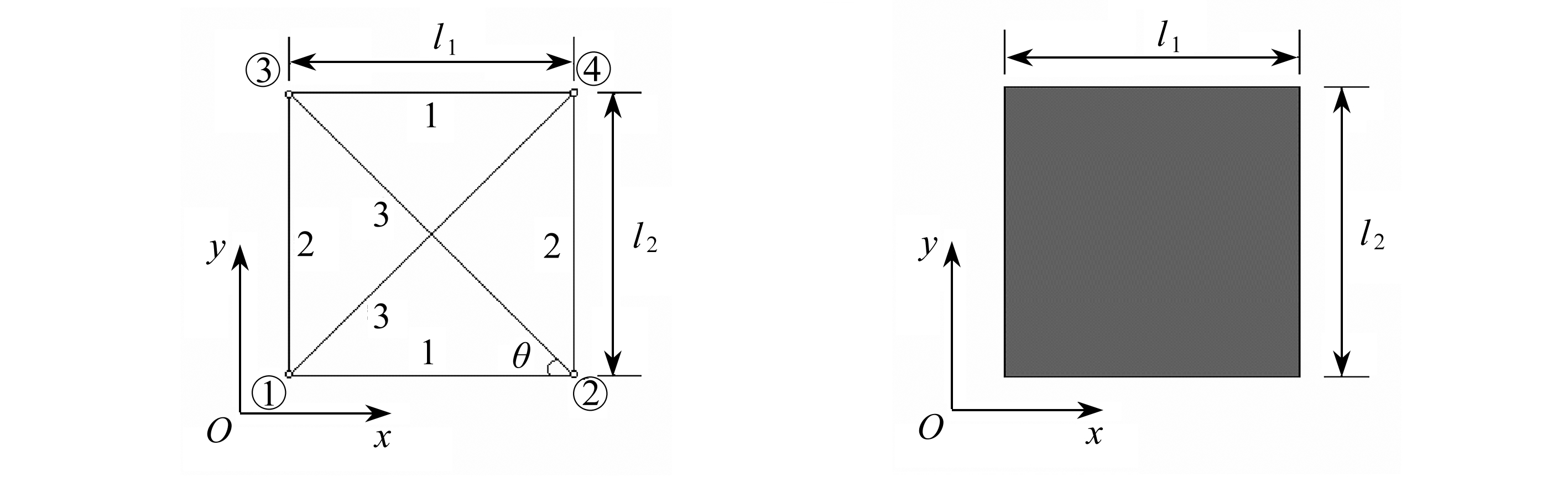(a) 温度场示意图 (b) 交界处温度变化
(a) Schematic diagram of the temperature field (b) Temperature variation at the interface

Fig. 2 Schematic diagram of the method

## 3 公 式 推 导

### 3.1第一层解析解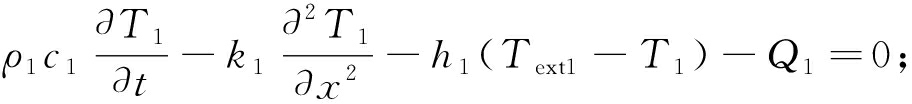(5)

x=0时(6)

x=l1

T1(l1,t)=f1,2(t)=v1,2t

(7)

T1(x,0)=Tina

(8)

T1(x,t)=v1(x,t)+w1(x,t)，

(9)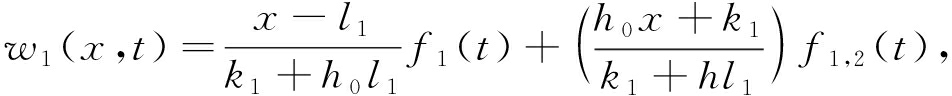(10)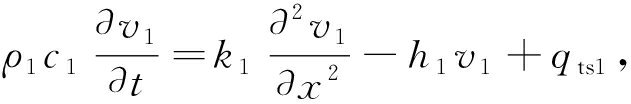(11)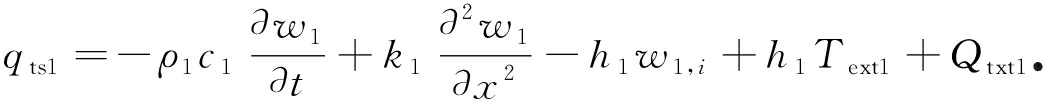(12)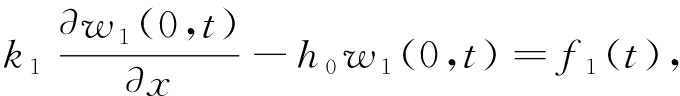(13)

w1(l1,t)=f1,2(t)

(14)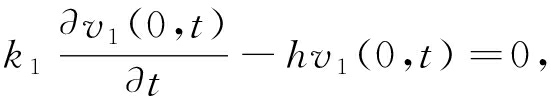(15)

v1(0,t)=0

(16)

v1(x,0)=Tina-w1(x,0)

(17)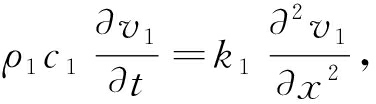(18)

v1(x,t)=Y1(x)G1(t)

(19)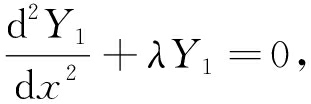(20)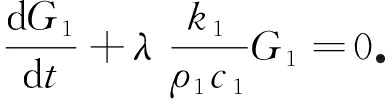(21)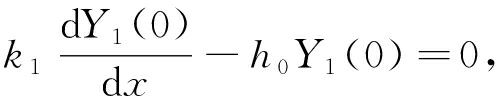(22)

Y1(l1)=0

(23)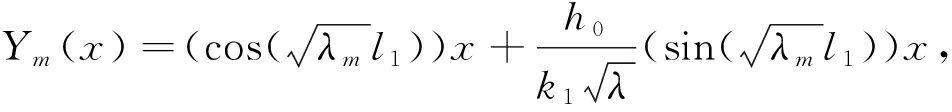(24)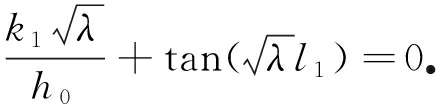(25)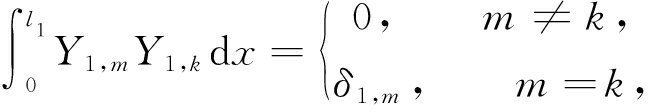(26)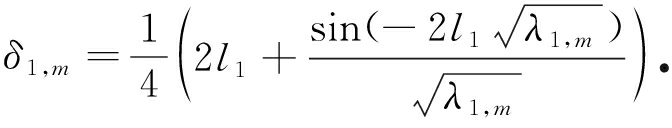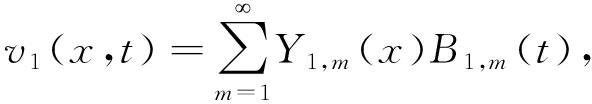(27)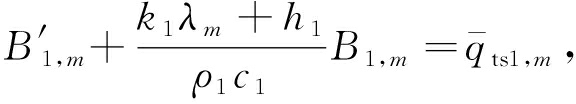(28)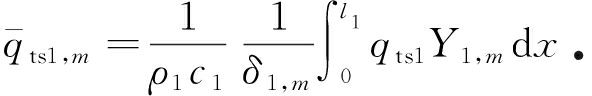(29)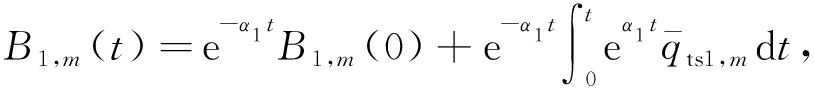(30)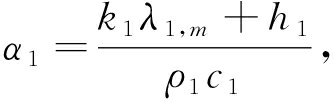(31)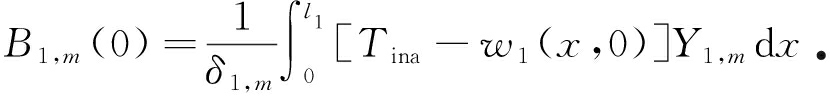(32)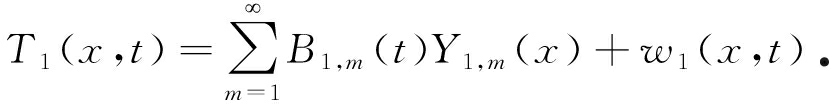(33)

### 3.2第n(n≥2)层解析解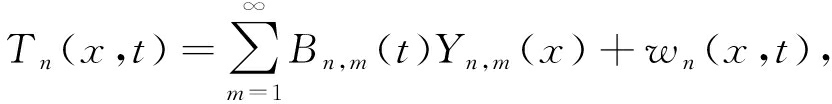(34)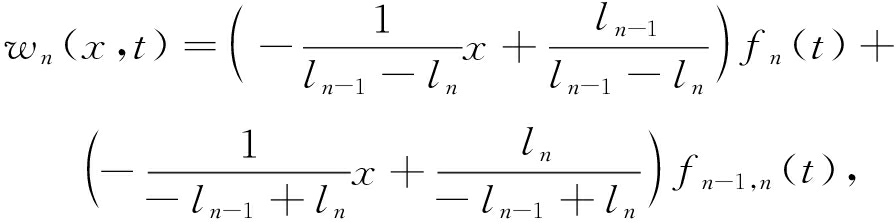(35)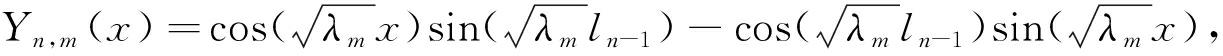(36)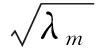为式(36)的正根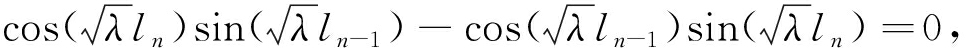(37)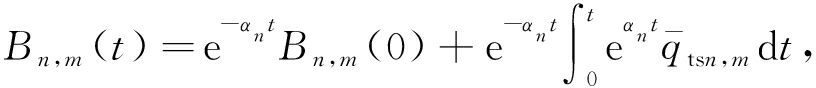(38)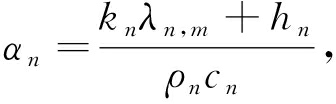(39)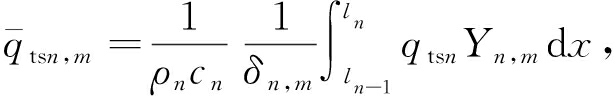(40)(41)

δn,m=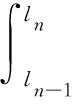Yn,mYn,kdx=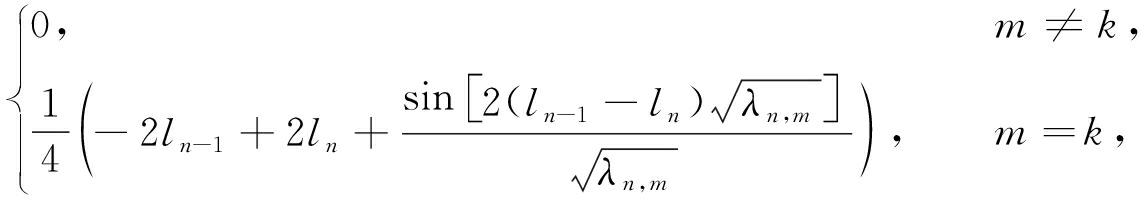(42)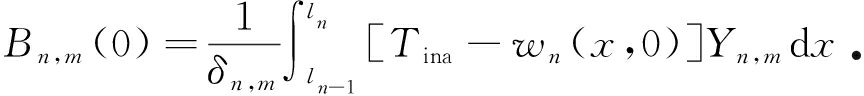(43)

### 3.3确定vn-1,n的值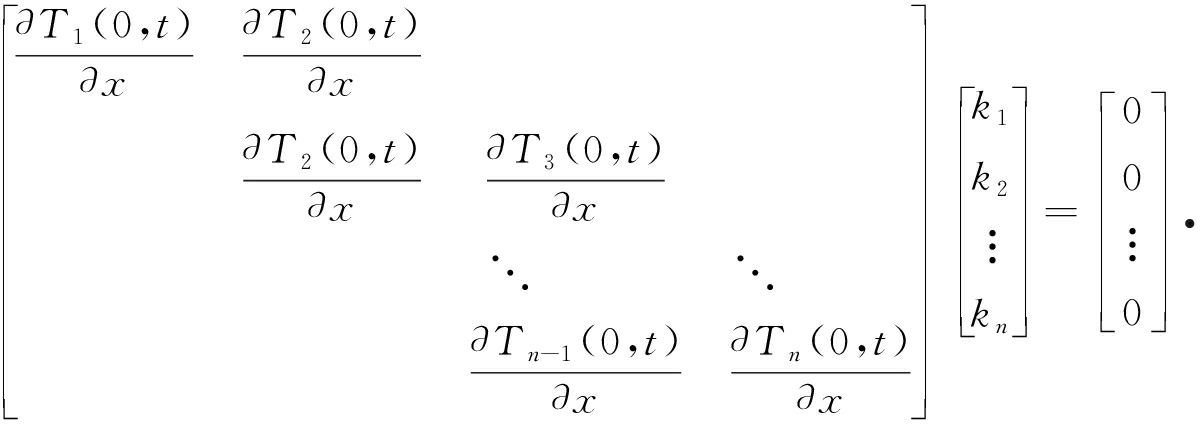(44)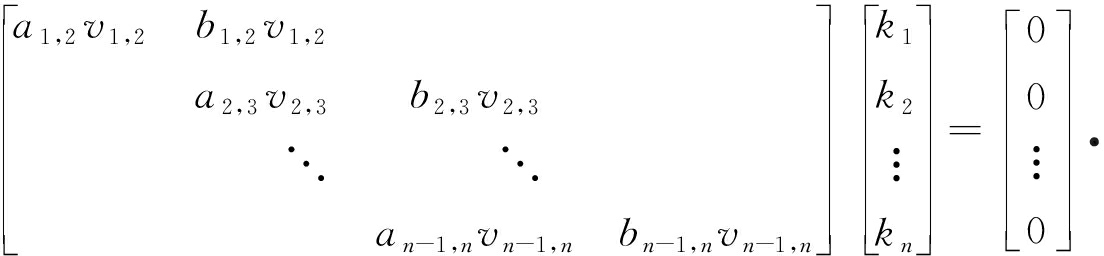(45)

## 4 算 例 分 析

Table 1 Parameters of the layered structure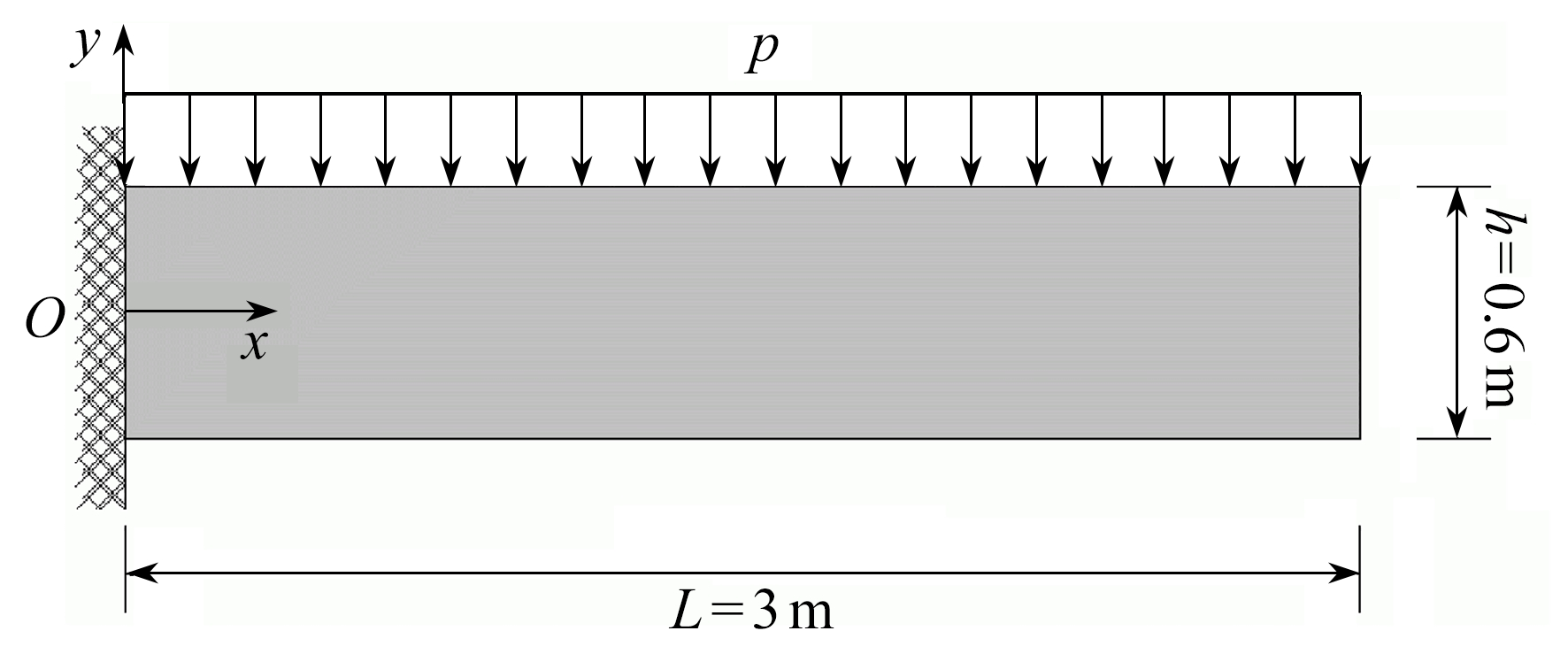Fig. 3 The temperature distribution along x-axis Fig. 4 The temperature variation at the structural surface

## 5 结 论

1) 基于微分思想，将层合材料交界处的温度变化曲线在微小时间段内近似为直线后利用分离变量法对各微小时间段的温度场进行了求解，进而求得了整个时间域内的温度场

2) 用本文的方法对某三层材料传热问题进行了分析，计算结果符合实际物理现象并且和有限元方法计算结果一致，进而验证了该方法的正确性

 WANG B L, CUI Y J. Transient inter laminar thermal stress in multi-layered thermoelectric materials[J]. Applied Thermal Engineering, 2017(2): 55-67.

 KUMAR D, KUMAR P, RAI K N. A study on DPL model of heat transfer in bi-layer tissues during MFH treatment[J]. Computers in Biology & Medicine, 2016, 75(2): 160-172.

 MATSUMOTO N, OKUNO T, OBARA H, et al. Multi-layer heat conduction analysis using SPH method[J]. Transactions of the Japan Society of Mechanical Engineers, 2016, 82(3): 77-82.

 LU S, LIU J, LIN G, et al. Modified scaled boundary finite element analysis of 3D steady-state heat conduction in anisotropic layered media[J]. International Journal of Heat & Mass Transfer, 2017, 108(1): 2462-2471.

 刘芳, 施卫平. 用格子Boltzmann方法模拟非线性热传导方程[J]. 应用数学和力学, 2015, 36(11): 1158-1166.(LIU Fang, SHI Weiping. Simulation of the nonlinear heat conduction equation with the lattice Boltzmann method[J]. Applied Mathematics and Mechanics, 2015, 36(11): 1158-1166.(in Chinese))

 詹涌强, 张传林. 解抛物型方程的一族高精度隐式差分格式[J]. 应用数学和力学, 2014, 35(7): 790-797.(ZHAN Yongqiang , ZHANG Chuanlin. A family of high accuracy implicit difference schemes for solving parabolic equations[J]. Applied Mathematics and Mechanics, 2014, 35(7): 790-797.(in Chinese))

 盛宏玉, 李和平, 叶建乔, 等. 介质热传导瞬态分析的一种新半数值解法[J]. 合肥工业大学学报(自然科学版), 2010, 33(5): 709-712.(SHENG Hongyu, LI Heping, YE Jianqiao, et al. A new semi-numerical approach for the transient heat conduction analysis of laminated medium[J]. Journal of Hefei University of Technology(Natural Science), 2010, 33(5): 709-712.(in Chinese))

 蓝林华, 富明慧, 刘祚秋. 结构瞬态热传导方程的一种精细解法[J]. 中山大学学报(自然科学版), 2011, 50(5): 1-6.(LAN Linhua, FU Minghui, LIU Zuoqiu. A precise solution of transient heat conduction equation for laminate structure[J]. Acta Scientiarum Naturalium Universitatis Sunyatseni, 2011, 50(5): 1-6.(in Chinese))

 李金娥, 王保林, 常冬梅. 材料的非傅里叶热传导及热应力[J]. 固体力学学报, 2011, 32(S1): 248-253.(LI Jin’e, WANG Baolin, CHANG Dongmei. Non-Fourier heat conduction and thermal stress of non-Fourier heat conduction an thermal stress of layered materials[J]. Chinese Journal of Solid Mechanics, 2011, 32(S1): 248-253.(in Chinese))

 富明慧, 陈焯智. 层合圆柱三维温度场分析的半解析-精细积分法[J]. 应用力学学报, 2012, 29(1): 15-20.(FU Minghui, CHEN Chuozhi. The semi-analytical precise integration method for the analysis of three dimensional temperature field of laminated cylinders[J]. Chinese Journal of Applied Mechanics, 2012, 29(1): 15-20.(in Chinese))

 LIU K C, WANG Y N, CHEN Y S. Investigation on the bio-heat transfer with the dual-phase-lag effect[J]. International Journal of Thermal Sciences, 2012, 58: 29-35.

 MOVAHEDIAN B, BOROOMAND B. The solution of direct and inverse transient heat conduction problems with layered materials using exponential basis functions[J]. International Journal of Thermal Sciences, 2014, 77: 186-198.

 MOHAJER M, AYANI M B, TABRIZI H B. Numerical study of non-Fourier heat conduction in a biolayer spherical living tissue during hyperthermia[J]. Journal of Thermal Biology, 2016, 62: 181-185.

 XU F, LU T J, SEFFEN K A. Bio-thermomechanics of skin tissues[J]. Journal of the Mechanics & Physics of Solids, 2008, 56(5): 1852-1884.

 LIN S M, LI C Y. Analytical solutions of non-Fourier bio-heat conductions for skin subjected to pulsed laser heating[J]. International Journal of Thermal Sciences, 2016, 110: 146-158.

 LIU K C, CHEN H T, CHENG P J. Inverse investigation of non-Fourier heat conduction in tissue[J]. Journal of Thermal Biology, 2016, 21(2): 68-73.

LI Xixia, DAI Haiyan, LI Changyu. A new method for solving heat transfer problems of laminate materials based on the differential theory[J]. Applied Mathematics and Mechanics, 2018, 39(6): 728-736.

# A New Method for Solving Heat Transfer Problems of Laminate Materials Based on the Differential Theory①

LI Xixia, DAI Haiyan, LI Changyu

(Guangzhou College of South China University of Technology, Guangzhou 510800, P.R.China)

Abstract: A new analytical method for solving heat transfer problems of laminate materials was proposed based on the differential theory. The curves of temperature variation at the interface of the laminate material were approximately linear in a small time interval. With the method of separation of variables, the analytical solution containing unknown coefficients of each layer in a small time interval was obtained. According to the continuous condition of energy at the interface, the unknown coefficients of each layer were determined. Then the temperature field in the whole time domain was obtained. Finally, the analytical method was used to solve the heat transfer problem of a 3-layer structure. The analytical results were compared with those of the finite element method, and the influences of several parameters on the temperature field were discussed, with the correctness of the proposed method verified.

Key words: laminate material; heat conduction; separation of variables; differential theory

DOI: 10.21656/1000-0887.380116

*收稿日期 2017-05-02； 修订日期： 2017-07-17

ⓒ 应用数学和力学编委会，ISSN 1000-0887